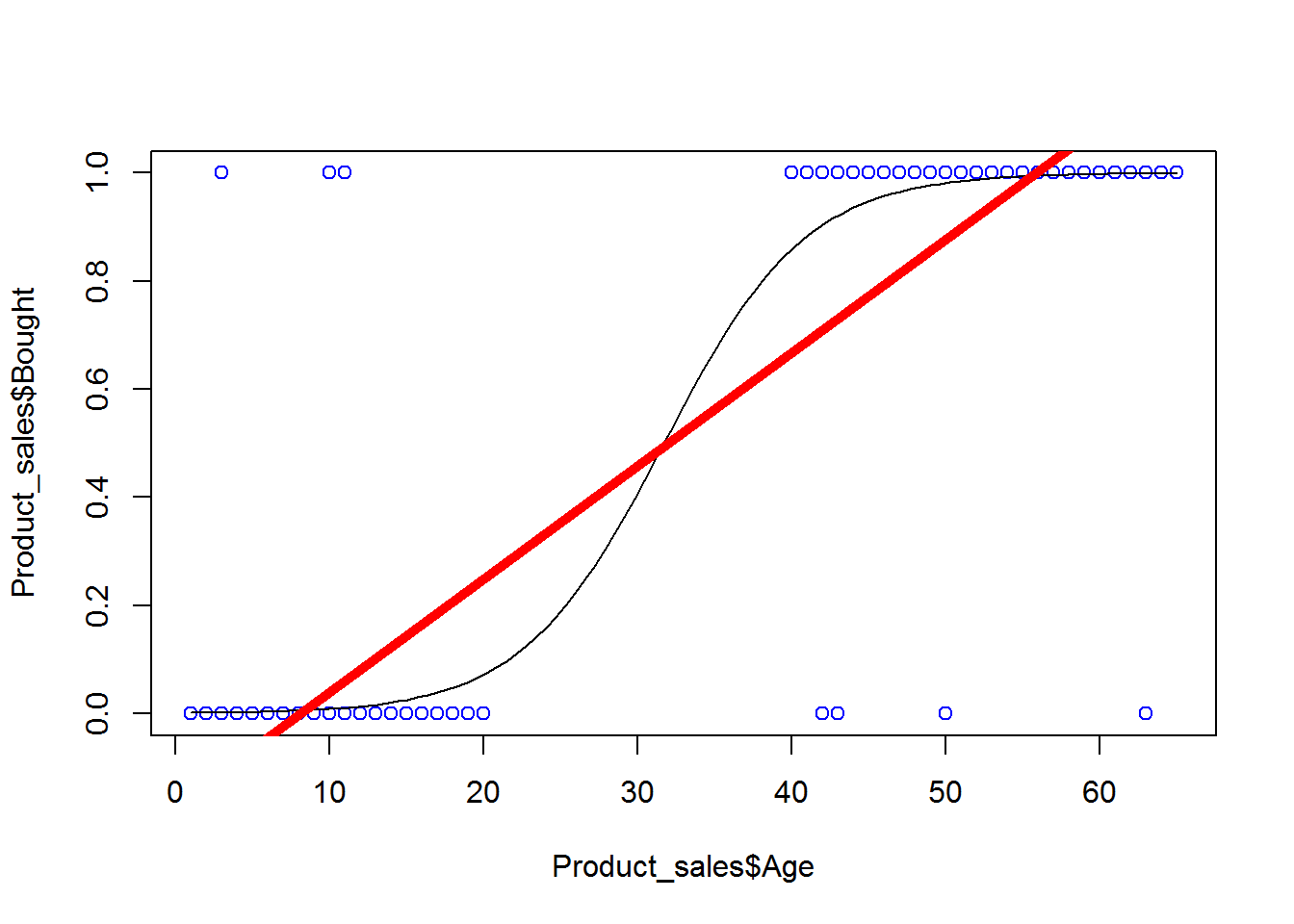• No products in the cart.

# 203.2.2 Logistic Function to Regression

### Logistic Regression Function

In previous section, we studied about Logistic Regression, why do we need it?

• Logistic regression models the logit of the outcome, instead of the outcome i.e. instead of winning or losing, we build a model for log odds of winning or losing
• Natural logarithm of the odds of the outcome
• ln(Probability of the outcome (p)/Probability of not having the outcome (1-p))

$P(y|x) = \frac{e^{(\beta_0+ \beta_1X)}}{1+e^{(\beta_0+ \beta_1X)}}$

### Lab: Logistic Regression

1. Import Dataset: Product Sales Data/Product_sales.csv
1. Build a logistic Regression line between Age and buying
1. A 25 years old customer, will he buy the product?
1. If Age is 105 then will that customer buy the product?
1. Draw a scatter plot between Age and Buy. Include both linear and logistic regression lines on the same chart.

### Logistic Regression in R

1. Import Dataset: Product Sales Data/Product_sales.csv
Product_sales<- read.csv("C:\\Amrita\\Datavedi\\Product Sales Data\\Product_sales.csv")
1. Build a logistic Regression line between Age and buying
prod_sales_Logit_model <- glm(Bought ~ Age,family=binomial(logit),data=Product_sales)
summary(prod_sales_Logit_model)
##
## Call:
## glm(formula = Bought ~ Age, family = binomial(logit), data = Product_sales)
##
## Deviance Residuals:
##     Min       1Q   Median       3Q      Max
## -3.6922  -0.1645  -0.0619   0.1246   3.5378
##
## Coefficients:
##             Estimate Std. Error z value Pr(>|z|)
## (Intercept) -6.90975    0.72755  -9.497   <2e-16 ***
## Age          0.21786    0.02091  10.418   <2e-16 ***
## ---
## Signif. codes:  0 '***' 0.001 '**' 0.01 '*' 0.05 '.' 0.1 ' ' 1
##
## (Dispersion parameter for binomial family taken to be 1)
##
##     Null deviance: 640.425  on 466  degrees of freedom
## Residual deviance:  95.015  on 465  degrees of freedom
## AIC: 99.015
##
## Number of Fisher Scoring iterations: 7
1. A 25 years old customer, will he buy the product?
new_data<-data.frame(Age=25)
predict(prod_sales_Logit_model,new_data,type="response")
##         1
## 0.1879529
1. If Age is 105 then will that customer buy the product?
new_data<-data.frame(Age=105)
predict(prod_sales_Logit_model,new_data,type="response")
##         1
## 0.9999999
1. Draw a scatter plot between Age and Buy. Include both linear and logistic regression lines on the same chart.
plot(Product_sales$Age,Product_sales$Bought,col = "blue")
abline(prod_sales_model, lwd = 5, col="red")The next post is about multiple logistic regression.

21st June 2017

### 0 responses on "203.2.2 Logistic Function to Regression"

Statinfer Software Solutions LLP

Software Technology Parks of India,
NH16, Krishna Nagar, Benz Circle,